# COMPLEX NUMBERS

Get a low cost access to TINACloud to edit the examples or create your own circuitsMESH AND LOOP CURRENT METHODS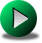PRINCIPLES OF ALTERNATING CURRENT

In this and the following chapters, we will present a very important topic: AC, or Alternating Current. The name alternating current is not very precise and normally covers circuits with sinusoidal voltages and currents; however, alternating current can also mean any arbitrary current waveform. The importance of AC voltage is that this kind of voltage is used for the main electric power source in homes and industry throughout the world. It is also the basis for many electronics, telecommunication, and industrial applications.

To handle sinusoidal waveforms and the circuits associated with them, we will use a simple and elegant method called the method of phasors. Phasors are based on the properties of complex numbers, which are ideal for representing sinusoidal quantities. In this chapter, we will summarize the principal facts about complex numbers and their operations. We will also show how TINAâ€™s Interpreter makes it easy to do calculations with complex numbers.

Complex numbers consist of two parts, a real part(x), which is a real number, and a so called imaginary part (y), which is a real number multiplied by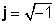, the imaginary unit. The complex number z, therefore, can be described as:

z = x + jy

where.

Examples of complex numbers:

z 1 = 1+ j

z 2 = 4-2 j

z 3 =3- 5j

Complex numbers were originally introduced in the seventeenth century to represent the roots of polynomials which could not be represented with real numbers alone. For example, the roots of the equation x2 +2x +2 = 0 can only be described as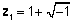and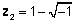, or using the notation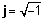, z1= 1+ j and z2= 1- j. Using the new notation to investigate the properties of expressions, mathematicians were able to prove theorems and solve problems which until then had been difficult if not impossible to solve. This led to the elaboration of complex algebra and complex functions, which now are widely used in mathematics and engineering.

Geometric representation of complex numbers

Rectangular form

Because a complex number can always be separated into its real and complex parts, we can represent a complex number as a point on a two-dimensional plane. The real part of a complex number is the projection of the point onto the real axis, and the imaginary part of the number is the projection onto the imaginary axis. When a complex number is represented as the sum of real and imaginary parts, we say it is in rectangular or algebraic form.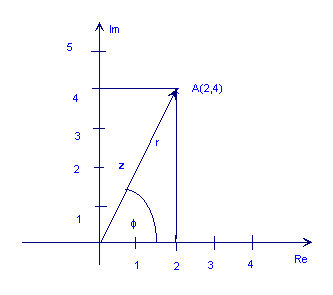The following figure shows the complex number z = 2 + 4j

Polar and exponential form

As you can see from the figure above, the point A could also be represented by the length of the arrow, r (also called the absolute value, magnitude, or amplitude), and its angle (or phase), Ď† relative in a counterclockwise direction to the positive horizontal axis. This is the polar form of a complex number. It is denoted as r ∠ Ď†.

The next step is very important. A complex number in polar form can also be written in exponential form: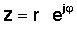This simple expression is distinctive in that it has an imaginary number in the exponent instead of the usual real number. This complex exponential behaves very differently from the exponential function with a real argument. While ex grows rapidly in magnitude for increasing x>0 and decreases for x<0, the function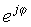has the same magnitude (z =1) for any Ď†. Furthermore, its complex values lie on the unit circle.

Eulerâ€™s formula provides a unifying link among the rectangular, polar, and exponential forms of complex numbers:

z = x + jy = r e jĎ† = r ( cos Ď† + j sin Ď† )

where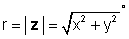and Ď† = tan-1 (y/x).

For our example above, z = 2 + 4j: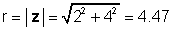Ď† = tan-1 ( 4/2) = 63.4Â°

therefore.

Or vice-versa: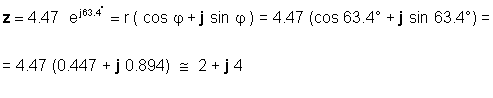You will need to be adept at using both forms, depending on the application. For example, addition or subtraction are obviously easier to do when the numbers are in rectangular form, while multiplication and division are easier to do when the numbers are in exponential form.

## Operations with complex numbers

The operations that can be done with complex numbers are similar to those for real numbers. The rules and some new definitions are summarized below.

### Operations with j

The operations with j simply follow from the definition of the imaginary unit,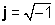To be able to work fast and accurately, you should memorize these rules:

j 2 = -1

j 3 =-j

j 4 =1

1/j = -j

Proof:

j2 = -1 simply follows from the definition of, since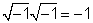For 1/j, we multiply 1/jby j / j =1 and get j/ (j j) = j /(-1) = -j.

## Complex conjugate

The complex conjugate of a complex number is easily derived and is quite important. To obtain the complex conjugate of a complex number in rectangular form, simply change the sign of the imaginary part. To do so for a number in exponential form, change the sign of the angle of the complex number while keeping its absolute value the same.

The complex conjugate of a complex number z is often denoted by z*.

Given the complex number z=a+ jb, its complex conjugate is z*=aâ€“ jb.

If z is given in exponential form,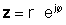, its complex conjugate isUsing the definitions above, it is easy to see that a complex number multiplied by its complex conjugate gives the square of the absolute value of the complex number:

z z* = r2 = a2 + b2

Also, by adding or subtracting any complex number and its conjugate, we get the following relations:

z + z * = 2a

therefore

Re(z) = a = ( z + z * )/ 2

Similarly:

z - z * =j2b

therefore

Im(z) = b = ( z -z * )/ 2j

Proof: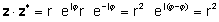or multiplying the real and imaginary parts and using j2= -1

z z* =(a+ jb)(a â€“ jb)=a2+a jb - a jb â€“ jbjb = a2j2= a2 + b2

z + z* =a+ jb + a â€“ jb = 2a

z - z*=a+ jb - a + jb =j2b

Numerical examples:

In rectangular form:

z =3+ j4

z* =3â€“ j4

z z * =9+16=25

In polar form

z = 5 ∠ 53.13Â°

z * =5 ∠- 53.13Â°

In exponential form: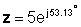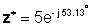Addition and subtraction

Addition and subtraction of complex numbers is straightforwardâ€”we only need to add the real and imaginary parts separately. For example, if

z1 = 3 â€“ 4j and z2 = 2 + 3j

then

z1 + z2 = 3 â€“ 4j + 2 + 3j = 3 + 2 â€“ 4j + 3j = 5 - j

z1 - z2 = 3 â€“ 4j - 2 - 3j = 3 - 2 â€“ 4j - 3j = 1 â€“ j7

Obviously, we should use the rectangular form for these operations. If the numbers are given in exponential or polar form, we should transform them first to rectangular form using Eulerâ€™s formula, as given earlier.

###### Multiplication

There are two methods for multiplication of complex numbers--

Multiplication of complex numbers given in rectangular form

To carry out the operation, simply multiply the real and imaginary parts of one number in turn by the real and imaginary parts of the other number and use the identity j2 = -1.

z1z2 = (a1 + jb1) (a2 + jb2)= a1 a2 + jb1a2+ jb2a1 â€“ b1b2 = a1 a2â€“ b1b2 + j(b1a2+ jb2a1)

When the complex numbers are given numerically, it is not necessary to use the formula above. For example, let

z1 = 3 â€“ 4j and z2 = 2 + 3j

With direct multiplication of the components:

z1z2 = (3 â€“ 4j)( 2 + 3j)=6- 8j +9j + 12 =18 + j

or using the formula: z1z2 = a1 a2â€“ b1b2 + j(b1a2+ b2a1)

z1z2 = 6 +12 + j ( -8 + 9) = 18 + j

We think youâ€™re more likely to make an error if you use the formula than if you multiply the components directly.

 {Solution by TINA's Interpreter} z1:=3-4*j z2:=2+3*j z1*z2=[18+1*j]

Multiplication of complex numbers given in polar or exponential form

To carry out this operation, multiply the absolute values and add the angles of the two complex numbers. Let: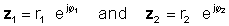Then using the rule of multiplication of exponential functions: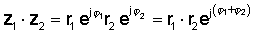or in polar form

z1 z2 = r1 r2 ∠ Ď†1 + Ď†2

Note: We have already used this rule when we calculated z z *above. Since the angle of the conjugate has the opposite sign of the original angle, a complex number multiplied by its own conjugate is always a real number; namely, the square of its absolute value: z z * = r2

For example, let:

z1 = 5 ∠ 30Â° and z2 = 4 ∠ -60Â°

then

z1z2 = 20 ∠ -30Â°

or in exponential formMultiplication is obviously simpler when the numbers are in polar or exponential form.

However, if the complex numbers are given in rectangular form, you should consider performing the multiplication directly as shown above, since there are additional steps if you convert the numbers to polar form before multiplying them. Another factor to consider is whether you want the answers to be in rectangular form or in polar/exponential form. For example, if the two numbers are in rectangular form but you would like their product in polar form, it makes sense to convert them immediately and then multiply them.

Division

There are two methods for division of complex numbers--

Division of complex numbers given in rectangular form

To carry out the operation, multiply the numerator and the denominator by the conjugate of the denominator. The denominator becomes a real number and the division is reduced to the multiplication of two complex numbers and a division by a real number, the square of the absolute value of the denominator.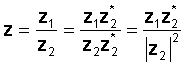For example let:

z1 = 3 â€“ 4j and z2 = 2 + 3j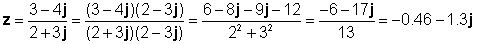Letâ€™s check this result with TINAâ€™s Interpreter:

 {Solution by TINA's Interpreter} z1:=3-4*j z2:=2+3*j z1/z2=[-461.5385m-1.3077*j]

Division of complex numbers given in polar or exponential form

To carry out the operation, divide the absolute values (magnitudes) and subtract the angle of the denominator from the angle of the numerator. Let:then using the rule of division of exponential functionsor in polar form

z 1 / z2 = r1 / r2 Ď† 1- Ď† 2

For example, let:

z 1 = 5 ∠ 30Â° and z 2 = 2 ∠ -60Â°

then

z 1 / z2 = 2.5 ∠ 90Â°

or in exponential and rectangular forms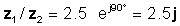Letâ€™s check this result with TINAâ€™s Interpreter:

 {Solution by TINA's Interpreter} z1:=5*exp(j*degtorad(30)) z2:=2*exp(j*degtorad(-60)) z1/z2=[0+2.5*j]

Division is obviously simpler when the numbers are in polar or exponential form.

However, if the complex numbers are given in rectangular form, you should consider performing the division directly using the complex conjugate method as shown above, since there are additional steps if you convert the numbers to polar form before dividing them. Another factor to consider is whether you want the answers to be in rectangular form or in polar/exponential form. For example, if the two numbers are in rectangular form, but you would like their quotient in polar form, it makes sense to convert them immediately and then divide them.

Now let us illustrate the use of complex numbers by more numerical problems. As usual, we will check our solutions using TINAâ€™s Interpreter. The interpreter works with radians, but it has standard functions for the conversion of radians to degrees or vice-versa.

Example 1 Find the polar representation:

z = 12 â€“ j 48or 49.48 ∠ - 75.96Â°

 {Solution by TINA's Interpreter} z:=12-j*48; abs(z)=[49.4773] arc(z)=[-1.3258] radtodeg(arc(z))=[-75.9638]

Example 2 Find the rectangular representation:

z = 25 e j 125 Â°{Solution by TINA's Interpreter} z:=25*exp(j*(degtorad(125))); z=[-14.3394+20.4788*j] Re(z)=[-14.3394] Im(z)=[20.4788]

Example 3 Find the polar representation of the following complex numbers:

z 1 = 12 + j 48 z2 = 12 - j48 z3= -12 + j 48 z4= -12 â€“ j 48

The absolute values of all four numbers are the same because the absolute value is independent of the signs. Only the angles are different.

 {Solution by TINA's Interpreter} z1:=12+j*48; abs(z1)=[49.4773] arc(z1)=[1.3258] radtodeg(arc(z1))=[75.9638] z2:=12-j*48; abs(z2)=[49.4773] arc(z2)=[-1.3258] radtodeg(arc(z2))=[-75.9638] z3:=-12+j*48; abs(z3)=[49.4773] arc(z3)=[1.8158] radtodeg(arc(z3))=[104.0362] z4:=-12-j*48: abs(z4)=[49.4773] arc(z4)=[-1.8158] radtodeg(arc(z4))=[-104.0362]

TINAâ€™s arc() function determines the angle of any complex number, automatically placing it correctly in one of the four quadrants.

Be careful, however, using the tan-1 function to find the angle, since it is restricted to returning angles only in the first and fourth quadrants (â€“90Â°<Ď†<90Â°).

Since z1 is located in the first quadrant of the coordinate system, the calculation is:

Î± 1 = tan-1(48/12)= tan-1(4)= 75.96Â°

Since z4 is located in the third quadrant of the coordinate system, tan-1does not returns the angle correctly. The angle calculation is:

Î± 4 = 180Â° +75.96Â° = 255.96Â° or -360Â° +255.96Â° = - 104.04Â° , which is the same as calculated by TINA.

z2 is located in the fourth quadrant of the coordinate system The angle calculation is:

Î± 2 = tan-1(-48/12)= tan-1(-4) = -75.96Â°

z3, however, is in the 2nd quadrant of the coordinate system, so tan-1 does not return the angle correctly. The angle calculation is:

Î± 3 = 180Â° -75.96Â° = 104.04Â°.

Example 4 We have two complex numbers: z1= 4 â€“ j 6 and z2 = 5 ej45 Â° .

Find z3 = z1 + z2; z4 = z1 - z2; z5 = z1 * z2; z6 = z1 / z2

First we solve the problem using TINAâ€™s Interpreter

 {Solution by TINA's Interpreter} z1:=4-j*6; z2:=5*exp(j*degtorad(45)); z3:=z1+z2; z3=[7.5355-2.4645*j] z4:=z1-z2; z4=[464.4661m-9.5355*j] z5:=z1*z2; z5=[35.3553-7.0711*j] z6:=z1/z2; z6=[-282.8427m-1.4142*j]

Notice how TINA effortlessly handles the two complex numbers given in different forms.

The solution is more complicated without the interpreter. So that we can compare the different methods of multiplication and division, we will first determine the polar form of z1 and the rectangular form of z2 .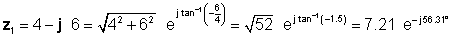Next, we find the four solutions using the easiest forms first: rectangular for addition and subtraction, and exponential for multiplication and division: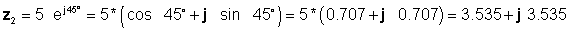z 3 = z1 + z2 = 4 â€“ j 6 + 3.535 + j 3.535 = 7.535 â€“ j2.465

z 4 = z1 â€“ z2 = 4 â€“ j 6 - 3.535 - j 3.535 = 0.465 â€“ j9.535

z 5 = z1 * z2 = 7.21*5*ej(-56.31 Â° +45Â° ) = 36.05 e â€“j11.31 Â° =36.03*(cos(-11.31Â° )+j*sin(-11.31Â° ))

z 5 = 35.33 â€“ j 7.07

z 6 = z1/z2= (7.21/5)*e j (-56.31 Â° -45Â° ) = 1.442 e â€“ j 101.31 Â° = 1.442 (cos(-101.31Â° )+j*sin(-101.31Â° ))

z 6 = -0.2828 â€“ j 1.414

which agree with the results obtained with the TINA Interpreter.

The multiplication carried out in rectangular form:

z 5 =z1*z2 = (4-j6)*3.535*(1+j) = 7.07*(2-j3)*(1+j)=7.07*(2-j3+j2+3)=7.07*(5-j)=35.35-j7.07

Finally the division carried out in rectangular form: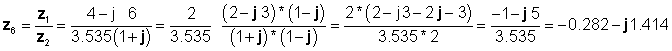which agree with the previous results.MESH AND LOOP CURRENT METHODSPRINCIPLES OF ALTERNATING CURRENT